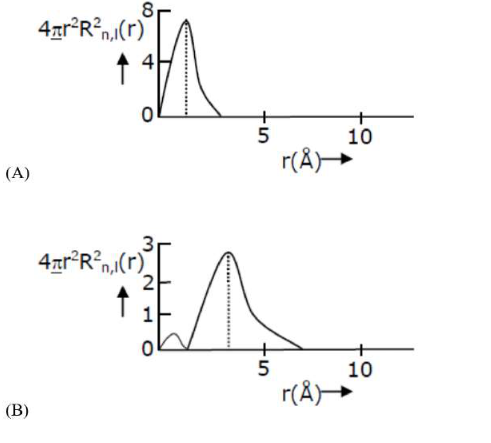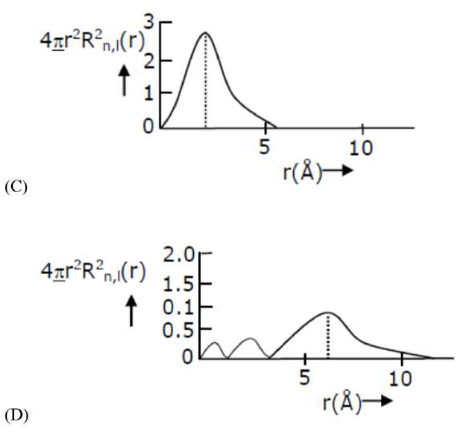# The plots of radial distribution functions for various orbitals of hydrogen atom against

Question:

The plots of radial distribution functions for various orbitals of hydrogen atom against ' $r$ ' are given below:The correct plot for $3 \mathrm{~s}$ orbital is:

1. D

2. B

3. A

4. C

Correct Option: 1

Solution:

$3 s$ orbital

Number of radial nodes $=\mathrm{n}-\ell-1$

For $3 \mathrm{~s}$ orbital $n=3 \quad \ell=0$

Number of radial nodes $=3-0-1=2$ It is correctly represented in graph of option $\mathrm{D}$